Aug 25, 2020

# Introduction

Although scRNA-seq has enabled us to characterize transcriptomes at single cell resolution to identify transcriptionally distinct cell-types and cell-states, it is a destructive protocol and thus only allows us to capture a static snapshot in time. As we are often interested in how cells change during dynamic processes such as differentiation, tumor development, drug response, etc, we would like to infer some information regarding directed temporal dynamics from this static snapshot. RNA velocity analysis is a computational approach that allows us to computationally predict where the cell is “heading” in terms of its gene expression (e.g. predict the future transcriptional state of cells). Details of RNA velocity analysis and modeling along with accompany software as part of the `velocyto.R` R package can be found in the original publication by La Manno et al., (2018). Recently, RNA velocity analysis was expanded by Bergen et al (2020) to further enable inference of gene-specific rates of transcription, splicing and degregation, accomodate transient cell-states, among other cool features and has been made available as part of the `scvelo` Python package.

I am personally much more familiar with R programming and generally prefer to stay within one programming language for reproducibility purposes. So rather than switching to Python to use `scvelo`, in this tutorial, I will demo the use `scvelo` from within R using R’s `reticulate` package.

# Setting up

First, we will need to install `reticulate`. It also helps to have Conda (https://docs.conda.io/en/latest/) installed to manage Python.

``````# install.packages("reticulate")
library(reticulate)
``````

I previously created a Conda environment called `r-velocity` and installed `scvelo` via:

``````# bash
pip install -U scvelo
``````

You can double check in Python that the install worked using:

``````# bash
conda activate r-velocity
python
>>> import scvelo as scv
``````

Now we can load up the appropriate Conda environment that has `scvelo` installed.

``````conda_list()
``````
``````##           name                                      python
## 1    anaconda3                   /opt/anaconda3/bin/python
## 2 r-reticulate /opt/anaconda3/envs/r-reticulate/bin/python
## 3   r-velocity   /opt/anaconda3/envs/r-velocity/bin/python
``````
``````use_condaenv("r-velocity", required = TRUE)
scv <- import("scvelo")
scv\$logging\$print_version()
``````
``````## Running scvelo 0.2.2 (python 3.8.5) on 2020-08-25 20:02.
``````

## scvelo

For demo purposes, we will work through the following tutorial (https://scvelo.readthedocs.io/) and work through how to interface with the results in R. We will download and use the built-in pancreas dataset.

``````adata <- scv\$datasets\$pancreas()
``````
``````## AnnData object with n_obs × n_vars = 3696 × 27998
##     obs: 'clusters_coarse', 'clusters', 'S_score', 'G2M_score'
##     var: 'highly_variable_genes'
##     uns: 'clusters_coarse_colors', 'clusters_colors', 'day_colors', 'neighbors', 'pca'
##     obsm: 'X_pca', 'X_umap'
##     layers: 'spliced', 'unspliced'
##     obsp: 'distances', 'connectivities'
``````

A UMAP embedding has already been generated and is accessible in the pancreas dataset’s AnnData object. The same goes for cluster annotations. We can plot results using `scvelo`’s plotting functions. Note, this will result in a pop-up window.

``````scv\$pl\$scatter(adata, legend_loc='lower left', size=60)
``````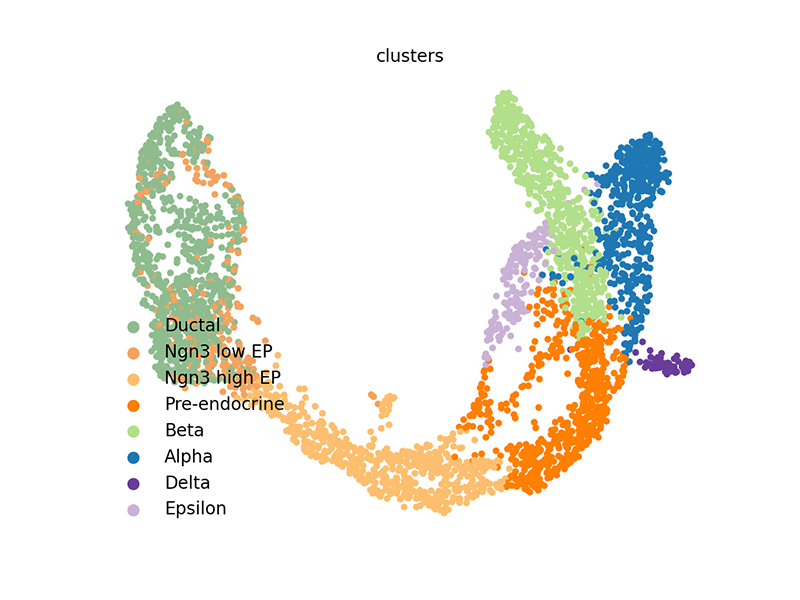We can also access these elements and plot them within R.

``````## get embedding

## get clusters, convert to colors
col <- rainbow(length(levels(clusters)), s=0.8, v=0.8)
cell.cols <- col[clusters]
names(cell.cols) <- names(clusters)

## simple plot
plot(emb, col=cell.cols, pch=16,
xlab='UMAP X', ylab='UMAP Y')
legend(x=-13, y=0,
legend=levels(clusters),
col=col,
pch=16)
``````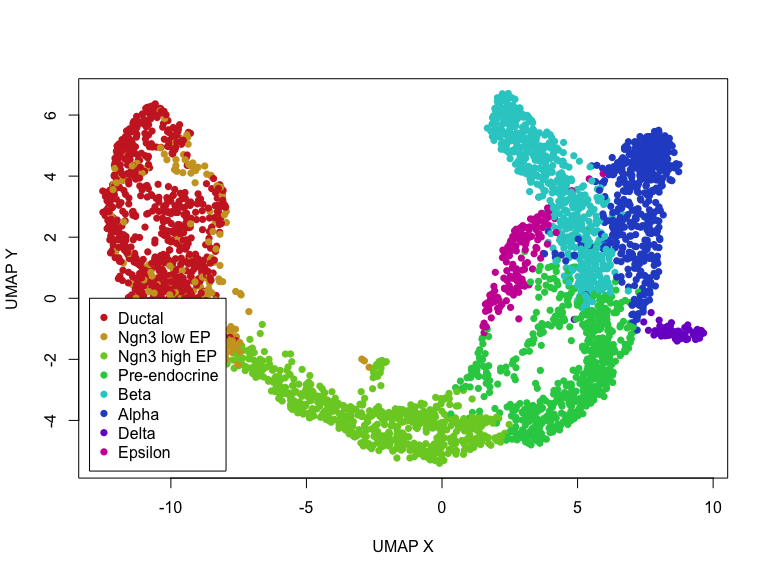Now, let’s actually run `scvelo`’s dynamic RNA velocity modeling on this pancreas data from within R! Note, that the main difference in terms of R function calls compared to python is now `.` are `\$`. Other than that, passing objects through `py_to_r` or `r_to_py` can fix a lot of errors.

``````## run scvelo dynamic model
scv\$pp\$moments(adata) ## normalize and compute moments

## takes awhile, so uncomment to save
``````

We can visualize the dynamic RNA velocities on the UMAP embedding using `scvelo`’s plotting functions. Again, this will result in a pop-up window.

``````## plot (creates pop up window)
## scv\$pl\$velocity_embedding_stream(adata, basis='pca') ## other embedding
``````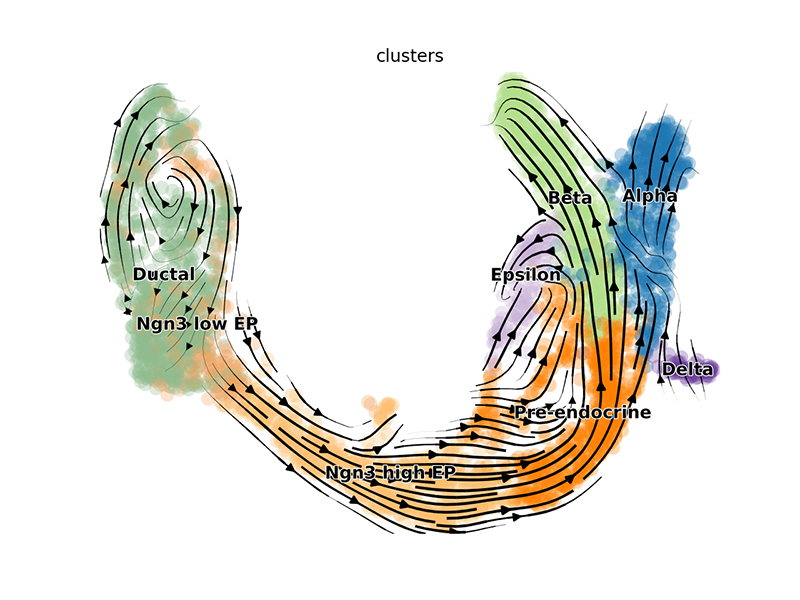We can also pull out the top genes driving the dynamic RNA velocities.

``````## top dynamic genes
topgenes_vals <- topgenes[,1]
names(topgenes_vals) <- rownames(topgenes)
topgenes_vals <- sort(topgenes_vals, decreasing=TRUE)
``````
``````##     Pcsk2     Top2a      Rps3     Gng12      Pak3       Ank
## 0.7811821 0.6639022 0.6355273 0.6131085 0.5956294 0.5955843
``````

We can further visualize their phase diagrams using `scvelo`’s plotting functions.

``````scv\$pl\$scatter(adata, basis=names(topgenes_vals)[1:5], ncols=5, frameon=FALSE)
``````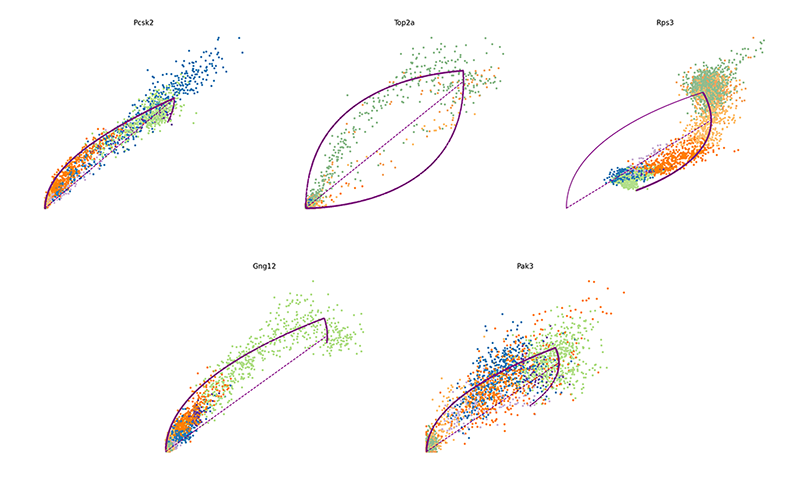## velocyto.R

By working within R, we can also easily compare results with the original, non-dynamic RNA velocity modeling with `velocyto.R`.

``````library(velocyto.R)
``````
``````## Loading required package: Matrix
``````
``````fit.quantile <- 0.1

## pull out spliced and unspliced matrices from AnnData
colnames(emat) <- colnames(nmat) <- cells
rownames(emat) <- rownames(nmat) <- genes

## pull out PCA
rownames(pcs) <- cells
cell.dist <- as.dist(1-cor(t(pcs))) ## cell distance in PC space

## filter genes
gexp1 <- log10(rowSums(emat)+1)
gexp2 <- log10(rowSums(nmat)+1)
#plot(gexp1, gexp2)
good.genes <- genes[gexp1 > 2 & gexp2 > 1]
``````

Now we can run the RNA velocity model using `velocyto.R`.

``````## velocyto model
rvel.cd <- gene.relative.velocity.estimates(emat[good.genes,], nmat[good.genes,],
deltaT=1, kCells=30,
cell.dist=cell.dist,
fit.quantile=fit.quantile,
mult=100)
## takes awhile, so uncomment to save
## save(rvel.cd, file="data/velocyto.RData")
``````

We can visualize the RNA velocities on the original UMAP embedding using `velocyto.R`’s plotting functions.

``````## Plot on embedding
show.velocity.on.embedding.cor(scale(emb), rvel.cd,
n = 100,
scale='sqrt',
cex=1, arrow.scale=1, show.grid.flow=TRUE,
min.grid.cell.mass=0.5, grid.n=30, arrow.lwd=2,
cell.colors=cell.cols)
``````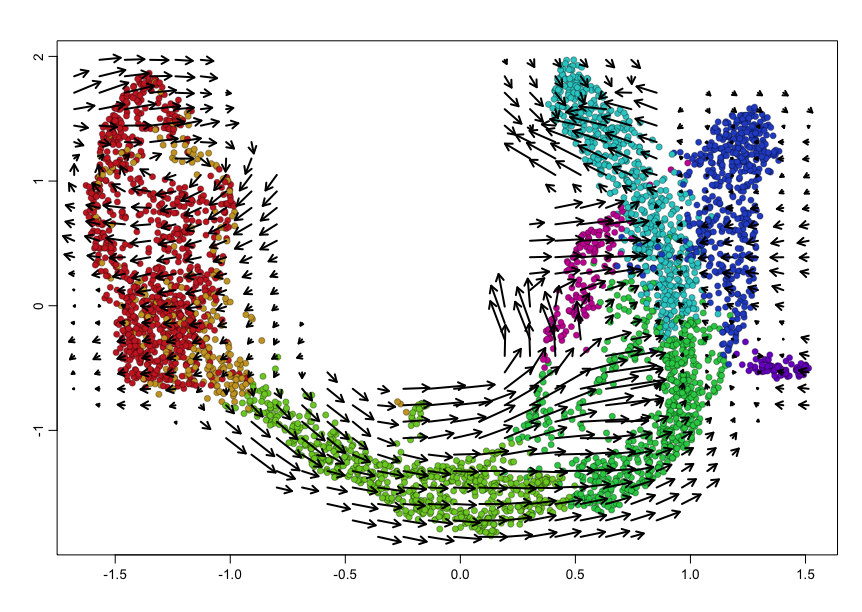We can also visualize phase diagrams for the top dynamic genes from `scvelo` using `velocyto.R`’s plotting functions.

``````## Gene plot
sapply(1:5, function(i) {
gene.relative.velocity.estimates(emat[good.genes,], nmat[good.genes,],
kCells = 30,
fit.quantile = fit.quantile,
old.fit = rvel.cd,
show.gene = names(topgenes_vals)[i],
cell.emb = emb,
cell.colors = cell.cols)
})
``````
``````## calculating convolved matrices ... done
``````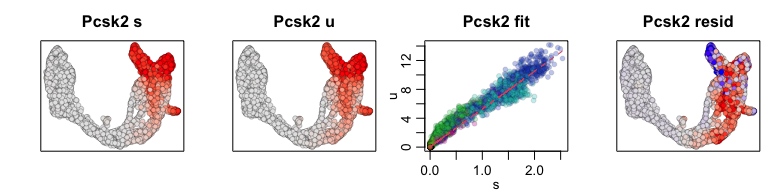``````## calculating convolved matrices ... done
``````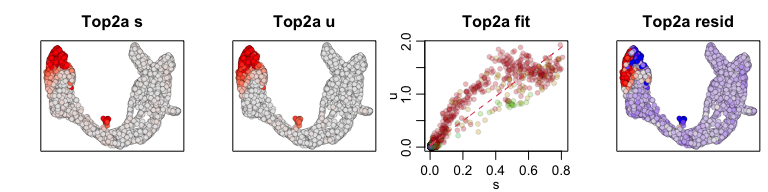``````## calculating convolved matrices ... done
``````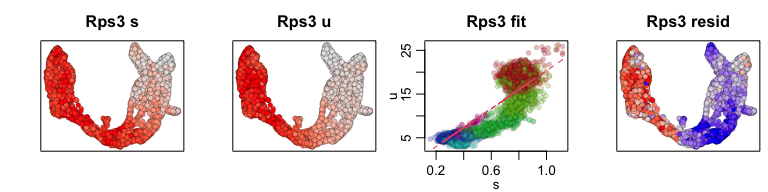``````## calculating convolved matrices ... done
``````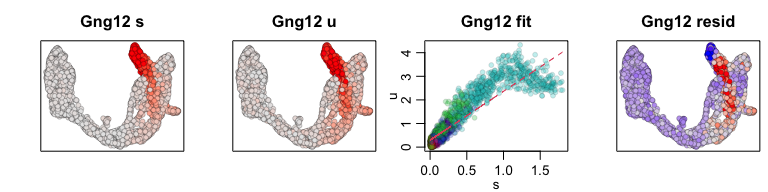``````## calculating convolved matrices ... done
``````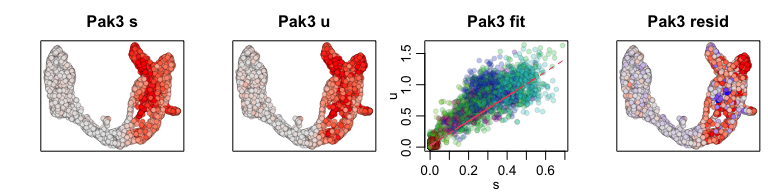Alternatively, if we wanted to use our own data, we can create an `AnnData` object such as follows. We can then use `scvelo` to run analyses. If you are more comfortable in R like me, a lot of filtering, clustering, and generating embeddings can be made within R and put into the `AnnData` object such that `scvelo` is only used for the dynamic RNA velocity component.

``````ad <- import("anndata", convert = FALSE)
dfobs <- data.frame(clusters, annotations)
rownames(dfobs) <- cells
dfvar <- data.frame(genes_attributes)
rownames(dfvar) <- genes
X=t(expression_matrix),
obs=dfobs,
var=dfvar,
layers=list('spliced'=t(emat), 'unspliced'=t(nmat)),
obsm=list('X_tsne'=emb, 'X_pca'=pcs\$x[,1:2])
)
``````

Try it out for yourself!

# Session info

``````sessionInfo()
``````
``````## R version 4.0.2 (2020-06-22)
## Platform: x86_64-apple-darwin17.0 (64-bit)
## Running under: macOS Catalina 10.15.6
##
## Matrix products: default
## BLAS:   /Library/Frameworks/R.framework/Versions/4.0/Resources/lib/libRblas.dylib
## LAPACK: /Library/Frameworks/R.framework/Versions/4.0/Resources/lib/libRlapack.dylib
##
## locale:
##  en_US.UTF-8/en_US.UTF-8/en_US.UTF-8/C/en_US.UTF-8/en_US.UTF-8
##
## attached base packages:
##  stats     graphics  grDevices utils     datasets  methods   base
##
## other attached packages:
##  velocyto.R_0.6  Matrix_1.2-18   reticulate_1.16
##
## loaded via a namespace (and not attached):
##   Rcpp_1.0.5          knitr_1.29          cluster_2.1.0
##   magrittr_1.5        BiocGenerics_0.34.0 splines_4.0.2
##   MASS_7.3-51.6       lattice_0.20-41     rlang_0.4.7
##  stringr_1.4.0       tools_4.0.2         parallel_4.0.2
##  grid_4.0.2          Biobase_2.48.0      nlme_3.1-148
##  mgcv_1.8-31         xfun_0.16           htmltools_0.5.0
##  yaml_2.2.1          digest_0.6.25       evaluate_0.14
##  rmarkdown_2.3       stringi_1.4.6       compiler_4.0.2
##  pcaMethods_1.80.0   jsonlite_1.7.0
``````